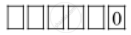CBSE (Arts) Class 11CBSE
Share

# How Many 6-digit Numbers Can Be Formed from the Digits 0, 1, 3, 5, 7 and 9 Which Are Divisible by 10 and No Digit is Repeated? - CBSE (Arts) Class 11 - Mathematics

ConceptConcept of Combinations

#### Question

How many 6-digit numbers can be formed from the digits 0, 1, 3, 5, 7 and 9 which are divisible by 10 and no digit is repeated?

#### Solution

A number is divisible by 10 if its units digits is 0.

Therefore, 0 is fixed at the units place.

Therefore, there will be as many ways as there are ways of filling 5 vacant placesin succession by the remaining 5 digits (i.e., 1, 3, 5, 7 and 9).

The 5 vacant places can be filled in 5! ways.

Hence, required number of 6-digit numbers = 5! = 120

Is there an error in this question or solution?

#### APPEARS IN

NCERT Solution for Mathematics Textbook for Class 11 (2018 to Current)
Chapter 7: Permutations and Combinations
Q: 5 | Page no. 157

#### Video TutorialsVIEW ALL 

Solution How Many 6-digit Numbers Can Be Formed from the Digits 0, 1, 3, 5, 7 and 9 Which Are Divisible by 10 and No Digit is Repeated? Concept: Concept of Combinations.
S